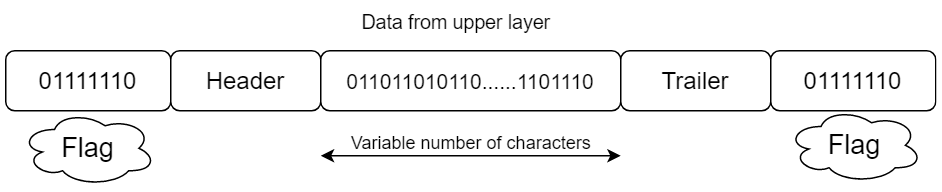# Bit-Stuffing in Java

Hello Tech Aspirants, I hope you are doing well. In this tutorial, we will learn the concept of bit stuffing in Java.
So, Data frames can contain an arbitrary number of bits which allows the character codes with an arbitrary number of bits at each character, Where each special frame begins and ends with a special bit pattern, let’s say (a flag byte). We know that when we are sending data in the payloads so at the beginning and the end we are having a flag byte which is .
Whenever the sender’s Data link layer encounters five consecutive 1’s in the data, it automatically stuffs a 0 bit into the outgoing bitstream which is called payloads.
If 6 1’s come then at the receiving end it might get misinterpreted that it is an end flag, So after the occurrence of each consecutive 5 1’s one zero will be stuffed & that’s why it is called Bit stuffing.

## Concept of bit stuffing in Java with codeThe above diagram is a frame in a bit-oriented protocol.

#### Explanation of Bit-Stuffing

When the receiver collapses with five consecutive incoming 1 bits, followed by a 0 bit, it automatically destuffs the 0 bit. Just as the concept of byte stuffing is completely transparent to the network layer, so is the bit stuffing.

#### Java Code for the above explanation

• Input d1 for the incoming destuffed string.
• Take a loop from 0 to d1.length(), if the characters don’t match with 0’s and 1’s break the loop and come out of the program.
• If the bit containing 1 (counter incrementing with 1 ) has not reached the 2nd if statement(counter=0)
, and the counter becomes exactly 5 stuff a  bit at the end of the current statement.
• Repeat the earlier point till the length of d1.
• Print the statements in the format
————————————
FLAG | STUFFED BIT | FLAG
————————————
• Flag–>01111110.
• Destuff the entire string by reversing the earlier statements.
• Print the destuffed bit.

```import java.util.*;
public class bit_stuffing
{
public static void main(String[] args)
{
Scanner sc=new Scanner(System.in);
System.out.print("Enter the message:-");
String d1 = sc.nextLine();
String remaining = new String();
String output=new String();
int counter = 0;
for(int i=0;i<d1.length();i++)
{

if (d1.charAt(i)!='1' && d1.charAt(i)!='0')
{
System.out.println("Enter valid Binary values");
return;
}
if(d1.charAt(i) == '1')
{
counter++;
remaining = remaining + d1.charAt(i);
}
else
{
remaining = remaining + d1.charAt(i);
counter = 0;
}
if(counter == 5)
{
remaining = remaining + '0';
counter = 0;
}
}
System.out.println("Flag--> 01111110");
String new1="|01111110 | "+remaining+" | 01111110|";
System.out.println("Stuffed data at intermediate site is:");
for(int k=0;k<=(28+d1.length());k++)
{
System.out.print("-");
}
System.out.println();
System.out.println(" "+new1);
for(int k=0;k<=(28+d1.length());k++)
{
System.out.print("-");
}
System.out.println();
counter=0;
for(int i=0;i<remaining.length();i++)
{

if(remaining.charAt(i) == '1')
{

counter++;
output = output + remaining.charAt(i);

}
else
{
output = output + remaining.charAt(i);
counter = 0;
}
if(counter == 5)
{
if((i+2)!=remaining.length())
{
output = output + remaining.charAt(i+2);
}
else
{
output=output + '1';
}
i=i+2;
counter = 1;
}
}
System.out.println("Destuffed BIT is: "+output);
}
}
```

As the output result, we will get what you can see below.

```Enter the message:-01111111110
Flag--> 01111110
Stuffed data at intermediate site is:
----------------------------------------
|01111110 | 011111011110 | 01111110|
----------------------------------------
Destuffed BIT is: 01111111110```

Hope this tutorial helped you.

1.Arjun says: